Calculus Volume 3

# 5.1Double Integrals over Rectangular Regions

Calculus Volume 35.1 Double Integrals over Rectangular Regions

### Learning Objectives

• 5.1.1 Recognize when a function of two variables is integrable over a rectangular region.
• 5.1.2 Recognize and use some of the properties of double integrals.
• 5.1.3 Evaluate a double integral over a rectangular region by writing it as an iterated integral.
• 5.1.4 Use a double integral to calculate the area of a region, volume under a surface, or average value of a function over a plane region.

In this section we investigate double integrals and show how we can use them to find the volume of a solid over a rectangular region in the $xyxy$-plane. Many of the properties of double integrals are similar to those we have already discussed for single integrals.

### Volumes and Double Integrals

We begin by considering the space above a rectangular region R. Consider a continuous function $f(x,y)≥0f(x,y)≥0$ of two variables defined on the closed rectangle R:

$R=[a,b]×[c,d]={(x,y)∈ℝ2|a≤x≤b,c≤y≤d}R=[a,b]×[c,d]={(x,y)∈ℝ2|a≤x≤b,c≤y≤d}$

Here $[a,b]×[c,d][a,b]×[c,d]$ denotes the Cartesian product of the two closed intervals $[a,b][a,b]$ and $[c,d].[c,d].$ It consists of rectangular pairs $(x,y)(x,y)$ such that $a≤x≤ba≤x≤b$ and $c≤y≤d.c≤y≤d.$ The graph of $ff$ represents a surface above the $xyxy$-plane with equation $z=f(x,y)z=f(x,y)$ where $zz$ is the height of the surface at the point $(x,y).(x,y).$ Let $SS$ be the solid that lies above $RR$ and under the graph of $ff$ (Figure 5.2). The base of the solid is the rectangle $RR$ in the $xyxy$-plane. We want to find the volume $VV$ of the solid $S.S.$

Figure 5.2 The graph of $f(x,y)f(x,y)$ over the rectangle $RR$ in the $xyxy$-plane is a curved surface.

We divide the region $RR$ into small rectangles $Rij,Rij,$ each with area $ΔAΔA$ and with sides $ΔxΔx$ and $ΔyΔy$ (Figure 5.3). We do this by dividing the interval $[a,b][a,b]$ into $mm$ subintervals and dividing the interval $[c,d][c,d]$ into $nn$ subintervals. Hence $Δx=b−am,Δx=b−am,$ $Δy=d−cn,Δy=d−cn,$ and $ΔA=ΔxΔy.ΔA=ΔxΔy.$

Figure 5.3 Rectangle $RR$ is divided into small rectangles $Rij,Rij,$ each with area $ΔA.ΔA.$

The volume of a thin rectangular box above $RijRij$ is $f(xij*,yij*)ΔA,f(xij*,yij*)ΔA,$ where $(xij*,yij*)(xij*,yij*)$ is an arbitrary sample point in each $RijRij$ as shown in the following figure.

Figure 5.4 A thin rectangular box above $RijRij$ with height $f(xij*,yij*).f(xij*,yij*).$

Using the same idea for all the subrectangles, we obtain an approximate volume of the solid $SS$ as $V≈∑i=1m∑j=1nf(xij*,yij*)ΔA.V≈∑i=1m∑j=1nf(xij*,yij*)ΔA.$ This sum is known as a double Riemann sum and can be used to approximate the value of the volume of the solid. Here the double sum means that for each subrectangle we evaluate the function at the chosen point, multiply by the area of each rectangle, and then add all the results.

As we have seen in the single-variable case, we obtain a better approximation to the actual volume if m and n become larger.

$V=limm,n→∞∑i=1m∑j=1nf(xij*,yij*)ΔAorV=limΔx,Δy→0∑i=1m∑j=1nf(xij*,yij*)ΔA.V=limm,n→∞∑i=1m∑j=1nf(xij*,yij*)ΔAorV=limΔx,Δy→0∑i=1m∑j=1nf(xij*,yij*)ΔA.$

Note that the sum approaches a limit in either case and the limit is the volume of the solid with the base R. Now we are ready to define the double integral.

### Definition

The double integral of the function $f(x,y)f(x,y)$ over the rectangular region $RR$ in the $xyxy$-plane is defined as

$∬Rf(x,y)dA=limm,n→∞∑i=1m∑j=1nf(xi*,yj*)ΔA.∬Rf(x,y)dA=limm,n→∞∑i=1m∑j=1nf(xi*,yj*)ΔA.$
(5.1)

If $f(x,y)≥0,f(x,y)≥0,$ then the volume V of the solid S, which lies above $RR$ in the $xyxy$-plane and under the graph of f, is the double integral of the function $f(x,y)f(x,y)$ over the rectangle $R.R.$ If the function is ever negative, then the double integral can be considered a “signed” volume in a manner similar to the way we defined net signed area in The Definite Integral.

### Example 5.1

#### Setting up a Double Integral and Approximating It by Double Sums

Consider the function $z=f(x,y)=3x2−yz=f(x,y)=3x2−y$ over the rectangular region $R=[0,2]×[0,2]R=[0,2]×[0,2]$ (Figure 5.5).

1. Set up a double integral for finding the value of the signed volume of the solid S that lies above $RR$ and “under” the graph of $f.f.$
2. Divide R into four squares with $m=n=2,m=n=2,$ and choose the sample point as the upper right corner point of each square $(1,1),(2,1),(1,2),(1,1),(2,1),(1,2),$ and $(2,2)(2,2)$ (Figure 5.6) to approximate the signed volume of the solid S that lies above $RR$ and “under” the graph of $f.f.$
3. Divide R into four squares with $m=n=2,m=n=2,$ and choose the sample point as the midpoint of each square: $(1/2,1/2),(3/2,1/2),(1/2,3/2),and(3/2,3/2)(1/2,1/2),(3/2,1/2),(1/2,3/2),and(3/2,3/2)$ to approximate the signed volume.
Figure 5.5 The function $z=f(x,y)z=f(x,y)$ graphed over the rectangular region $R=[0,2]×[0,2].R=[0,2]×[0,2].$

#### Analysis

Notice that the approximate answers differ due to the choices of the sample points. In either case, we are introducing some error because we are using only a few sample points. Thus, we need to investigate how we can achieve an accurate answer.

### Checkpoint5.1

Use the same function $z=f(x,y)=3x2−yz=f(x,y)=3x2−y$ over the rectangular region $R=[0,2]×[0,2].R=[0,2]×[0,2].$

Divide R into the same four squares with $m=n=2,m=n=2,$ and choose the sample points as the upper left corner point of each square $(0,1),(1,1),(0,2),(0,1),(1,1),(0,2),$ and $(1,2)(1,2)$ (Figure 5.6) to approximate the signed volume of the solid S that lies above $RR$ and “under” the graph of $f.f.$

Note that we developed the concept of double integral using a rectangular region R. This concept can be extended to any general region. However, when a region is not rectangular, the subrectangles may not all fit perfectly into R, particularly if the base area is curved. We examine this situation in more detail in the next section, where we study regions that are not always rectangular and subrectangles may not fit perfectly in the region R. Also, the heights may not be exact if the surface $z=f(x,y)z=f(x,y)$ is curved. However, the errors on the sides and the height where the pieces may not fit perfectly within the solid S approach 0 as m and n approach infinity. Also, the double integral of the function $z=f(x,y)z=f(x,y)$ exists provided that the function $ff$ is not too discontinuous. If the function is bounded and continuous over R except on a finite number of smooth curves, then the double integral exists and we say that $ff$ is integrable over R.

Since $ΔA=ΔxΔy=ΔyΔx,ΔA=ΔxΔy=ΔyΔx,$ we can express $dAdA$ as $dxdydxdy$ or $dydx.dydx.$ This means that, when we are using rectangular coordinates, the double integral over a region $RR$ denoted by $∬Rf(x,y)dA∬Rf(x,y)dA$ can be written as $∬Rf(x,y)dxdy∬Rf(x,y)dxdy$ or $∬Rf(x,y)dydx.∬Rf(x,y)dydx.$

Now let’s list some of the properties that can be helpful to compute double integrals.

### Properties of Double Integrals

The properties of double integrals are very helpful when computing them or otherwise working with them. We list here six properties of double integrals. Properties 1 and 2 are referred to as the linearity of the integral, property 3 is the additivity of the integral, property 4 is the monotonicity of the integral, and property 5 is used to find the bounds of the integral. Property 6 is used if $f(x,y)f(x,y)$ is a product of two functions $g(x)g(x)$ and $h(y).h(y).$

### Theorem5.1

#### Properties of Double Integrals

Assume that the functions $f(x,y)f(x,y)$ and $g(x,y)g(x,y)$ are integrable over the rectangular region R; S and T are subregions of R; and assume that m and M are real numbers.

1. The sum $f(x,y)+g(x,y)f(x,y)+g(x,y)$ is integrable and
$∬R[f(x,y)+g(x,y)]dA=∬Rf(x,y)dA+∬Rg(x,y)dA.∬R[f(x,y)+g(x,y)]dA=∬Rf(x,y)dA+∬Rg(x,y)dA.$
2. If c is a constant, then $cf(x,y)cf(x,y)$ is integrable and
$∬Rcf(x,y)dA=c∬Rf(x,y)dA.∬Rcf(x,y)dA=c∬Rf(x,y)dA.$
3. If $R=S∪TR=S∪T$ and $S∩T=∅S∩T=∅$ except an overlap on the boundaries, then
$∬Rf(x,y)dA=∬Sf(x,y)dA+∬Tf(x,y)dA.∬Rf(x,y)dA=∬Sf(x,y)dA+∬Tf(x,y)dA.$
4. If $f(x,y)≥g(x,y)f(x,y)≥g(x,y)$ for $(x,y)(x,y)$ in $R,R,$ then
$∬Rf(x,y)dA≥∬Rg(x,y)dA.∬Rf(x,y)dA≥∬Rg(x,y)dA.$
5. If $m≤f(x,y)≤M,m≤f(x,y)≤M,$ then
$m×A(R)≤∬Rf(x,y)dA≤M×A(R).m×A(R)≤∬Rf(x,y)dA≤M×A(R).$
6. In the case where $f(x,y)f(x,y)$ can be factored as a product of a function $g(x)g(x)$ of $xx$ only and a function $h(y)h(y)$ of $yy$ only, then over the region $R={(x,y)|a≤x≤b,c≤y≤d},R={(x,y)|a≤x≤b,c≤y≤d},$ the double integral can be written as
$∬Rf(x,y)dA=(∫abg(x)dx)(∫cdh(y)dy).∬Rf(x,y)dA=(∫abg(x)dx)(∫cdh(y)dy).$

These properties are used in the evaluation of double integrals, as we will see later. We will become skilled in using these properties once we become familiar with the computational tools of double integrals. So let’s get to that now.

### Iterated Integrals

So far, we have seen how to set up a double integral and how to obtain an approximate value for it. We can also imagine that evaluating double integrals by using the definition can be a very lengthy process if we choose larger values for $mm$ and $n.n.$ Therefore, we need a practical and convenient technique for computing double integrals. In other words, we need to learn how to compute double integrals without employing the definition that uses limits and double sums.

The basic idea is that the evaluation becomes easier if we can break a double integral into single integrals by integrating first with respect to one variable and then with respect to the other. The key tool we need is called an iterated integral.

### Definition

Assume $a,b,c,a,b,c,$ and $dd$ are real numbers. We define an iterated integral for a function $f(x,y)f(x,y)$ over the rectangular region $RR$ $=[a,b]×[c,d]=[a,b]×[c,d]$ as

1. $∫ab∫cdf(x,y)dydx=∫ab[∫cdf(x,y)dy]dx∫ab∫cdf(x,y)dydx=∫ab[∫cdf(x,y)dy]dx$
(5.2)

2. $∫cd∫abf(x,y)dxdy=∫cd[∫abf(x,y)dx]dy.∫cd∫abf(x,y)dxdy=∫cd[∫abf(x,y)dx]dy.$
(5.3)

The notation $∫ab[∫cdf(x,y)dy]dx∫ab[∫cdf(x,y)dy]dx$ means that we integrate $f(x,y)f(x,y)$ with respect to y while holding x constant. Similarly, the notation $∫cd[∫abf(x,y)dx]dy∫cd[∫abf(x,y)dx]dy$ means that we integrate $f(x,y)f(x,y)$ with respect to x while holding y constant. The fact that double integrals can be split into iterated integrals is expressed in Fubini’s theorem. Think of this theorem as an essential tool for evaluating double integrals.

### Theorem5.2

#### Fubini’s Theorem

Suppose that $f(x,y)f(x,y)$ is a function of two variables that is continuous over a rectangular region $R={(x,y)∈ℝ2|a≤x≤b,c≤y≤d}.R={(x,y)∈ℝ2|a≤x≤b,c≤y≤d}.$ Then we see from Figure 5.7 that the double integral of $ff$ over the region equals an iterated integral,

$∬Rf(x,y)dA=∬Rf(x,y)dxdy=∫ab∫cdf(x,y)dydx=∫cd∫abf(x,y)dxdy.∬Rf(x,y)dA=∬Rf(x,y)dxdy=∫ab∫cdf(x,y)dydx=∫cd∫abf(x,y)dxdy.$

More generally, Fubini’s theorem is true if $ff$ is bounded on $RR$ and $ff$ is discontinuous only on a finite number of continuous curves. In other words, $ff$ has to be integrable over $R.R.$

Figure 5.7 (a) Integrating first with respect to $yy$ and then with respect to $xx$ to find the area $A(x)A(x)$ and then the volume V; (b) integrating first with respect to $xx$ and then with respect to $yy$ to find the area $A(y)A(y)$ and then the volume V.

### Example 5.2

#### Using Fubini’s Theorem

Use Fubini’s theorem to compute the double integral $∬Rf(x,y)dA∬Rf(x,y)dA$ where $f(x,y)=xf(x,y)=x$ and $R=[0,2]×[0,1].R=[0,2]×[0,1].$

The double integration in this example is simple enough to use Fubini’s theorem directly, allowing us to convert a double integral into an iterated integral. Consequently, we are now ready to convert all double integrals to iterated integrals and demonstrate how the properties listed earlier can help us evaluate double integrals when the function $f(x,y)f(x,y)$ is more complex. Note that the order of integration can be changed (see Example 5.7).

### Example 5.3

#### Illustrating Properties i and ii

Evaluate the double integral $∬R(xy−3xy2)dA∬R(xy−3xy2)dA$ where $R={(x,y)|0≤x≤2,1≤y≤2}.R={(x,y)|0≤x≤2,1≤y≤2}.$

### Example 5.4

#### Illustrating Property v.

Over the region $R={(x,y)|1≤x≤3,1≤y≤2},R={(x,y)|1≤x≤3,1≤y≤2},$ we have $2≤x2+y2≤13.2≤x2+y2≤13.$ Find a lower and an upper bound for the integral $∬R(x2+y2)dA.∬R(x2+y2)dA.$

### Example 5.5

#### Illustrating Property vi

Evaluate the integral $∬ReycosxdA∬ReycosxdA$ over the region $R={(x,y)|0≤x≤π2,0≤y≤1}.R={(x,y)|0≤x≤π2,0≤y≤1}.$

### Checkpoint5.2

1. Use the properties of the double integral and Fubini’s theorem to evaluate the integral
$∫01∫−13(3−x+4y)dydx.∫01∫−13(3−x+4y)dydx.$
2. Show that $0≤∬RsinπxcosπydA≤1320≤∬RsinπxcosπydA≤132$ where $R=(0,14)(14,12).R=(0,14)(14,12).$

As we mentioned before, when we are using rectangular coordinates, the double integral over a region $RR$ denoted by $∬Rf(x,y)dA∬Rf(x,y)dA$ can be written as $∬Rf(x,y)dxdy∬Rf(x,y)dxdy$ or $∬Rf(x,y)dydx.∬Rf(x,y)dydx.$ The next example shows that the results are the same regardless of which order of integration we choose.

### Example 5.6

#### Evaluating an Iterated Integral in Two Ways

Let’s return to the function $f(x,y)=3x2−yf(x,y)=3x2−y$ from Example 5.1, this time over the rectangular region $R=[0,2]×[0,3].R=[0,2]×[0,3].$ Use Fubini’s theorem to evaluate $∬Rf(x,y)dA∬Rf(x,y)dA$ in two different ways:

1. First integrate with respect to y and then with respect to x;
2. First integrate with respect to x and then with respect to y.

#### Analysis

With either order of integration, the double integral gives us an answer of 15. We might wish to interpret this answer as a volume in cubic units of the solid $SS$ below the function $f(x,y)=3x2−yf(x,y)=3x2−y$ over the region $R=[0,2]×[0,3].R=[0,2]×[0,3].$ However, remember that the interpretation of a double integral as a (non-signed) volume works only when the integrand $ff$ is a nonnegative function over the base region $R.R.$

### Checkpoint5.3

Evaluate $∫y=−3y=2∫x=3x=5(2−3x2+y2)dxdy.∫y=−3y=2∫x=3x=5(2−3x2+y2)dxdy.$

In the next example we see that it can actually be beneficial to switch the order of integration to make the computation easier. We will come back to this idea several times in this chapter.

### Example 5.7

#### Switching the Order of Integration

Consider the double integral $∬Rxsin(xy)dA∬Rxsin(xy)dA$ over the region $R={(x,y)|0≤x≤3,0≤y≤2}R={(x,y)|0≤x≤3,0≤y≤2}$ (Figure 5.8).

1. Express the double integral in two different ways.
2. Analyze whether evaluating the double integral in one way is easier than the other and why.
3. Evaluate the integral.
Figure 5.8 The function $z=f(x,y)=xsin(xy)z=f(x,y)=xsin(xy)$ over the rectangular region $R=[0,3]×[0,2].R=[0,3]×[0,2].$

### Checkpoint5.4

Evaluate the integral $∬RxexydA∬RxexydA$ where $R=[0,1]×[0,ln5].R=[0,1]×[0,ln5].$

### Applications of Double Integrals

Double integrals are very useful for finding the area of a region bounded by curves of functions. We describe this situation in more detail in the next section. However, if the region is a rectangular shape, we can find its area by integrating the constant function $f(x,y)=1f(x,y)=1$ over the region $R.R.$

### Definition

The area of the region $RR$ is given by $A(R)=∬R1dA.A(R)=∬R1dA.$

This definition makes sense because using $f(x,y)=1f(x,y)=1$ and evaluating the integral make it a product of length and width. Let’s check this formula with an example and see how this works.

### Example 5.8

#### Finding Area Using a Double Integral

Find the area of the region $R={(x,y)|0≤x≤3,0≤y≤2}R={(x,y)|0≤x≤3,0≤y≤2}$ by using a double integral, that is, by integrating 1 over the region $R.R.$

We have already seen how double integrals can be used to find the volume of a solid bounded above by a function $f(x,y)f(x,y)$ over a region $RR$ provided $f(x,y)≥0f(x,y)≥0$ for all $(x,y)(x,y)$ in $R.R.$ Here is another example to illustrate this concept.

### Example 5.9

#### Volume of an Elliptic Paraboloid

Find the volume $VV$ of the solid $SS$ that is bounded by the elliptic paraboloid $2x2+y2+z=27,2x2+y2+z=27,$ the planes $x=3x=3$ and $y=3,y=3,$ and the three coordinate planes.

### Checkpoint5.5

Find the volume of the solid bounded above by the graph of $f(x,y)=xysin(x2y)f(x,y)=xysin(x2y)$ and below by the $xyxy$-plane on the rectangular region $R=[0,1]×[0,π].R=[0,1]×[0,π].$

Recall that we defined the average value of a function of one variable on an interval $[a,b][a,b]$ as

$fave=1b−a∫abf(x)dx.fave=1b−a∫abf(x)dx.$

Similarly, we can define the average value of a function of two variables over a region R. The main difference is that we divide by an area instead of the width of an interval.

### Definition

The average value of a function of two variables over a region $RR$ is

$fave=1AreaR∬Rf(x,y)dA.fave=1AreaR∬Rf(x,y)dA.$
(5.4)

In the next example we find the average value of a function over a rectangular region. This is a good example of obtaining useful information for an integration by making individual measurements over a grid, instead of trying to find an algebraic expression for a function.

### Example 5.10

#### Calculating Average Storm Rainfall

The weather map in Figure 5.10 shows an unusually moist storm system associated with the remnants of Hurricane Karl, which dumped 4–8 inches (100–200 mm) of rain in some parts of the Midwest on September 22–23, 2010. The area of rainfall measured 300 miles east to west and 250 miles north to south. Estimate the average rainfall over the entire area in those two days.

Figure 5.10 Effects of Hurricane Karl, which dumped 4–8 inches (100–200 mm) of rain in some parts of southwest Wisconsin, southern Minnesota, and southeast South Dakota over a span of 300 miles east to west and 250 miles north to south.

### Checkpoint5.6

A contour map is shown for a function $f(x,y)f(x,y)$ on the rectangle $R=[−3,6]×[−1,4].R=[−3,6]×[−1,4].$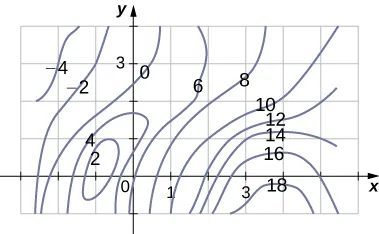1. Use the midpoint rule with $m=3m=3$ and $n=2n=2$ to estimate the value of $∬Rf(x,y)dA.∬Rf(x,y)dA.$
2. Estimate the average value of the function $f(x,y).f(x,y).$

### Section 5.1 Exercises

In the following exercises, use the midpoint rule with $m=4m=4$ and $n=2n=2$ to estimate the volume of the solid bounded by the surface $z=f(x,y),z=f(x,y),$ the vertical planes $x=1,x=1,$ $x=2,x=2,$ $y=1,y=1,$ and $y=2,y=2,$ and the horizontal plane $z=0.z=0.$

1.

$f ( x , y ) = 4 x + 2 y + 8 x y f ( x , y ) = 4 x + 2 y + 8 x y$

2.

$f ( x , y ) = 16 x 2 + y 2 f ( x , y ) = 16 x 2 + y 2$

In the following exercises, estimate the volume of the solid under the surface $z=f(x,y)z=f(x,y)$ and above the rectangular region R by using a Riemann sum with $m=n=2m=n=2$ and the sample points to be the lower left corners of the subrectangles of the partition.

3.

$f(x,y)=sinx−cosy,f(x,y)=sinx−cosy,$ $R=[0,π]×[0,π]R=[0,π]×[0,π]$

4.

$f(x,y)=cosx+cosy,f(x,y)=cosx+cosy,$ $R=[0,π]×[0,π2]R=[0,π]×[0,π2]$

5.

Use the midpoint rule with $m=n=2m=n=2$ to estimate $∬Rf(x,y)dA,∬Rf(x,y)dA,$ where the values of the function f on $R=[8,10]×[9,11]R=[8,10]×[9,11]$ are given in the following table.

 y x 9 9.5 10 10.5 11 8 9.8 5 6.7 5 5.6 8.5 9.4 4.5 8 5.4 3.4 9 8.7 4.6 6 5.5 3.4 9.5 6.7 6 4.5 5.4 6.7 10 6.8 6.4 5.5 5.7 6.8
6.

The values of the function f on the rectangle $R=[0,2]×[7,9]R=[0,2]×[7,9]$ are given in the following table. Estimate the double integral $∬Rf(x,y)dA∬Rf(x,y)dA$ by using a Riemann sum with $m=n=2.m=n=2.$ Select the sample points to be the upper right corners of the subsquares of R.

 $y0=7y0=7$ $y1=8y1=8$ $y2=9y2=9$ $x0=0x0=0$ 10.22 10.21 9.85 $x1=1x1=1$ 6.73 9.75 9.63 $x2=2x2=2$ 5.62 7.83 8.21
7.

The depth of a children’s 4-ft by 4-ft swimming pool, measured at 1-ft intervals, is given in the following table.

1. Estimate the volume of water in the swimming pool by using a Riemann sum with $m=n=2.m=n=2.$ Select the sample points using the midpoint rule on $R=[0,4]×[0,4].R=[0,4]×[0,4].$
2. Approximate the average depth of the swimming pool.
 y x 0 1 2 3 4 0 1 1.5 2 2.5 3 1 1 1.5 2 2.5 3 2 1 1.5 1.5 2.5 3 3 1 1 1.5 2 2.5 4 1 1 1 1.5 2
8.

The depth of a 3-ft by 3-ft hole in the ground, measured at 1-ft intervals, is given in the following table.

1. Estimate the volume of the hole by using a Riemann sum with $m=n=3m=n=3$ and the sample points to be the upper left corners of the subsquares of R.
2. Approximate the average depth of the hole.
 y x 0 1 2 3 0 6 6.5 6.4 6 1 6.5 7 7.5 6.5 2 6.5 6.7 6.5 6 3 6 6.5 5 5.6
9.

The level curves $f(x,y)=kf(x,y)=k$ of the function f are given in the following graph, where k is a constant.

1. Apply the midpoint rule with $m=n=2m=n=2$ to estimate the double integral $∬Rf(x,y)dA,∬Rf(x,y)dA,$ where $R=[0.2,1]×[0,0.8].R=[0.2,1]×[0,0.8].$
2. Estimate the average value of the function f on R.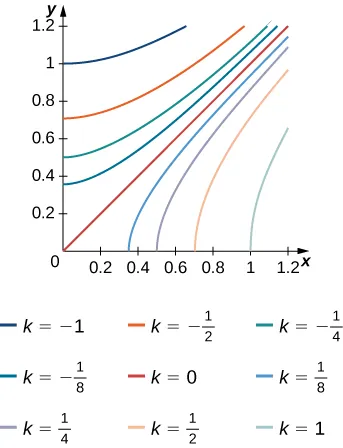10.

The level curves $f(x,y)=kf(x,y)=k$ of the function f are given in the following graph, where k is a constant.

1. Apply the midpoint rule with $m=n=2m=n=2$ to estimate the double integral $∬Rf(x,y)dA,∬Rf(x,y)dA,$ where $R=[0.1,0.5]×[0.1,0.5].R=[0.1,0.5]×[0.1,0.5].$
2. Estimate the average value of the function f on R.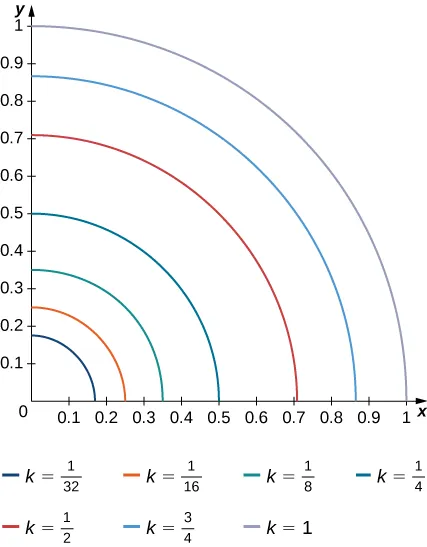11.

The solid lying under the surface $z=4−y2z=4−y2$ and above the rectangular region $R=[0,2]×[0,2]R=[0,2]×[0,2]$ is illustrated in the following graph. Evaluate the double integral $∬Rf(x,y)dA,∬Rf(x,y)dA,$ where $f(x,y)=4−y2,f(x,y)=4−y2,$ by finding the volume of the corresponding solid.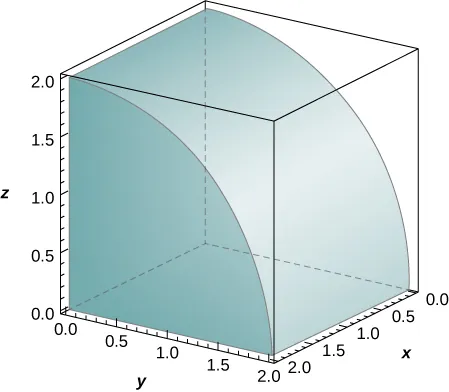12.

The solid lying under the plane $z=y+4z=y+4$ and above the rectangular region $R=[0,2]×[0,4]R=[0,2]×[0,4]$ is illustrated in the following graph. Evaluate the double integral $∬Rf(x,y)dA,∬Rf(x,y)dA,$ where $f(x,y)=y+4,f(x,y)=y+4,$ by finding the volume of the corresponding solid.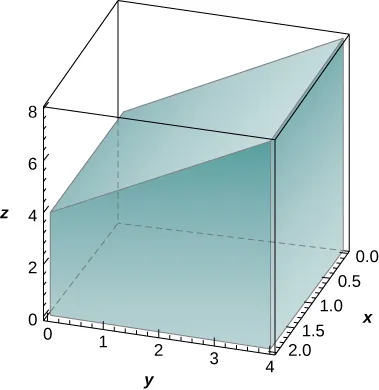In the following exercises, calculate the integrals by interchanging the order of integration.

13.

$∫ −1 1 ( ∫ −2 2 ( 2 x + 3 y + 5 ) d x ) d y ∫ −1 1 ( ∫ −2 2 ( 2 x + 3 y + 5 ) d x ) d y$

14.

$∫ 0 2 ( ∫ 0 1 ( x + 2 e y − 3 ) d x ) d y ∫ 0 2 ( ∫ 0 1 ( x + 2 e y − 3 ) d x ) d y$

15.

$∫ 1 27 ( ∫ 1 2 ( x 3 + y 3 ) d y ) d x ∫ 1 27 ( ∫ 1 2 ( x 3 + y 3 ) d y ) d x$

16.

$∫ 1 16 ( ∫ 1 8 ( x 4 + 2 y 3 ) d y ) d x ∫ 1 16 ( ∫ 1 8 ( x 4 + 2 y 3 ) d y ) d x$

17.

$∫ ln 2 ln 3 ( ∫ 0 l e x + y d y ) d x ∫ ln 2 ln 3 ( ∫ 0 l e x + y d y ) d x$

18.

$∫ 0 2 ( ∫ 0 1 3 x + y d y ) d x ∫ 0 2 ( ∫ 0 1 3 x + y d y ) d x$

19.

$∫ 1 6 ( ∫ 2 9 y x 2 d y ) d x ∫ 1 6 ( ∫ 2 9 y x 2 d y ) d x$

20.

$∫ 1 9 ( ∫ 4 2 x y 2 d y ) d x ∫ 1 9 ( ∫ 4 2 x y 2 d y ) d x$

In the following exercises, evaluate the iterated integrals by choosing the order of integration.

21.

$∫ 0 π ∫ 0 π / 2 sin ( 2 x ) cos ( 3 y ) d x d y ∫ 0 π ∫ 0 π / 2 sin ( 2 x ) cos ( 3 y ) d x d y$

22.

$∫ π / 12 π / 8 ∫ π / 4 π / 3 [ cot x + tan ( 2 y ) ] d x d y ∫ π / 12 π / 8 ∫ π / 4 π / 3 [ cot x + tan ( 2 y ) ] d x d y$

23.

$∫ 1 e ∫ 1 e [ 1 x sin ( ln x ) + 1 y cos ( ln y ) ] d x d y ∫ 1 e ∫ 1 e [ 1 x sin ( ln x ) + 1 y cos ( ln y ) ] d x d y$

24.

$∫ 1 e ∫ 1 e sin ( ln x ) cos ( ln y ) x y d x d y ∫ 1 e ∫ 1 e sin ( ln x ) cos ( ln y ) x y d x d y$

25.

$∫ 1 2 ∫ 1 2 ( ln y x + x 2 y + 1 ) d y d x ∫ 1 2 ∫ 1 2 ( ln y x + x 2 y + 1 ) d y d x$

26.

$∫ 1 e ∫ 1 2 x 2 ln ( x ) d y d x ∫ 1 e ∫ 1 2 x 2 ln ( x ) d y d x$

27.

$∫ 1 3 ∫ 1 2 y arctan ( 1 x ) d y d x ∫ 1 3 ∫ 1 2 y arctan ( 1 x ) d y d x$

28.

$∫ 0 1 ∫ 0 1 / 2 ( arcsin x + arcsin y ) d y d x ∫ 0 1 ∫ 0 1 / 2 ( arcsin x + arcsin y ) d y d x$

29.

$∫ 0 1 ∫ 1 2 x e x + 4 y d y d x ∫ 0 1 ∫ 1 2 x e x + 4 y d y d x$

30.

$∫ 1 2 ∫ 0 1 x e x − y d y d x ∫ 1 2 ∫ 0 1 x e x − y d y d x$

31.

$∫ 1 e ∫ 1 e ( ln y y + ln x x ) d y d x ∫ 1 e ∫ 1 e ( ln y y + ln x x ) d y d x$

32.

$∫ 1 e ∫ 1 e ( x ln y y + y ln x x ) d y d x ∫ 1 e ∫ 1 e ( x ln y y + y ln x x ) d y d x$

33.

$∫ 0 1 ∫ 1 2 ( x x 2 + y 2 ) d y d x ∫ 0 1 ∫ 1 2 ( x x 2 + y 2 ) d y d x$

34.

$∫ 0 1 ∫ 1 2 y x + y 2 d y d x ∫ 0 1 ∫ 1 2 y x + y 2 d y d x$

In the following exercises, find the average value of the function over the given rectangles.

35.

$f(x,y)=−x+2y,f(x,y)=−x+2y,$ $R=[0,1]×[0,1]R=[0,1]×[0,1]$

36.

$f(x,y)=x4+2y3,f(x,y)=x4+2y3,$ $R=[1,2]×[2,3]R=[1,2]×[2,3]$

37.

$f(x,y)=sinhx+sinhy,f(x,y)=sinhx+sinhy,$ $R=[0,1]×[0,2]R=[0,1]×[0,2]$

38.

$f(x,y)=arctan(xy),f(x,y)=arctan(xy),$ $R=[0,1]×[0,1]R=[0,1]×[0,1]$

39.

Let f and g be two continuous functions such that $0≤m1≤f(x)≤M10≤m1≤f(x)≤M1$ for any $x∈[a,b]x∈[a,b]$ and $0≤m2≤g(y)≤M20≤m2≤g(y)≤M2$ for any $y∈[c,d].y∈[c,d].$ Show that the following inequality is true:

$m 1 m 2 ( b − a ) ( c − d ) ≤ ∫ a b ∫ c d f ( x ) g ( y ) d y d x ≤ M 1 M 2 ( b − a ) ( c − d ) . m 1 m 2 ( b − a ) ( c − d ) ≤ ∫ a b ∫ c d f ( x ) g ( y ) d y d x ≤ M 1 M 2 ( b − a ) ( c − d ) .$

In the following exercises, use property v. of double integrals and the answer from the preceding exercise to show that the following inequalities are true.

40.

$1e2≤∬Re−x2−y2dA≤1,1e2≤∬Re−x2−y2dA≤1,$ where $R=[0,1]×[0,1]R=[0,1]×[0,1]$

41.

$π2144≤∬RsinxcosydA≤π248,π2144≤∬RsinxcosydA≤π248,$ where $R=[π6,π3]×[π6,π3]R=[π6,π3]×[π6,π3]$

42.

$0≤∬Re−ycosxdA≤π22,0≤∬Re−ycosxdA≤π22,$ where $R=[0,π2]×[0,π2]R=[0,π2]×[0,π2]$

43.

$0≤∬R(lnx)(lny)dA≤(e−1)2,0≤∬R(lnx)(lny)dA≤(e−1)2,$ where $R=[1,e]×[1,e]R=[1,e]×[1,e]$

44.

Let f and g be two continuous functions such that $0≤m1≤f(x)≤M10≤m1≤f(x)≤M1$ for any $x∈[a,b]x∈[a,b]$ and $0≤m2≤g(y)≤M20≤m2≤g(y)≤M2$ for any $y∈[c,d].y∈[c,d].$ Show that the following inequality is true:

$( m 1 + m 2 ) ( b − a ) ( c − d ) ≤ ∫ a b ∫ c d [ f ( x ) + g ( y ) ] d y d x ≤ ( M 1 + M 2 ) ( b − a ) ( c − d ) . ( m 1 + m 2 ) ( b − a ) ( c − d ) ≤ ∫ a b ∫ c d [ f ( x ) + g ( y ) ] d y d x ≤ ( M 1 + M 2 ) ( b − a ) ( c − d ) .$

In the following exercises, use property v. of double integrals and the answer from the preceding exercise to show that the following inequalities are true.

45.

$2e≤∬R(e−x2+e−y2)dA≤2,2e≤∬R(e−x2+e−y2)dA≤2,$ where $R=[0,1]×[0,1]R=[0,1]×[0,1]$

46.

$π236≤∬R(sinx+cosy)dA≤π2336,π236≤∬R(sinx+cosy)dA≤π2336,$ where $R=[π6,π3]×[π6,π3]R=[π6,π3]×[π6,π3]$

47.

$π2e−π/2≤∬R(cosx+e−y)dA≤π,π2e−π/2≤∬R(cosx+e−y)dA≤π,$ where $R=[0,π2]×[0,π2]R=[0,π2]×[0,π2]$

48.

$1e≤∬R(e−y−lnx)dA≤2,1e≤∬R(e−y−lnx)dA≤2,$ where $R=[0,1]×[0,1]R=[0,1]×[0,1]$

In the following exercises, the function f is given in terms of double integrals.

1. Determine the explicit form of the function f.
2. Find the volume of the solid under the surface $z=f(x,y)z=f(x,y)$ and above the region R.
3. Find the average value of the function f on R.
4. Use a computer algebra system (CAS) to plot $z=f(x,y)z=f(x,y)$ and $z=favez=fave$ in the same system of coordinates.
49.

[T] $f(x,y)=∫0y∫0x(xs+yt)dsdt,f(x,y)=∫0y∫0x(xs+yt)dsdt,$ where $(x,y)∈R=[0,1]×[0,1](x,y)∈R=[0,1]×[0,1]$

50.

[T] $f(x,y)=∫0x∫0y[cos(s)+cos(t)]dtds,f(x,y)=∫0x∫0y[cos(s)+cos(t)]dtds,$ where $(x,y)∈R=[0,3]×[0,3](x,y)∈R=[0,3]×[0,3]$

51.

Show that if f and g are continuous on $[a,b][a,b]$ and $[c,d],[c,d],$ respectively, then

$∫ a b ∫ c d [ f ( x ) + g ( y ) ] d y d x = ( d − c ) ∫ a b f ( x ) d x ∫ a b ∫ c d [ f ( x ) + g ( y ) ] d y d x = ( d − c ) ∫ a b f ( x ) d x$

$+ ∫ a b ∫ c d g ( y ) d y d x = ( b − a ) ∫ c d g ( y ) d y + ∫ c d ∫ a b f ( x ) d x d y . + ∫ a b ∫ c d g ( y ) d y d x = ( b − a ) ∫ c d g ( y ) d y + ∫ c d ∫ a b f ( x ) d x d y .$

52.

Show that $∫ab∫cdyf(x)+xg(y)dydx=12(d2−c2)(∫abf(x)dx)+12(b2−a2)(∫cdg(y)dy).∫ab∫cdyf(x)+xg(y)dydx=12(d2−c2)(∫abf(x)dx)+12(b2−a2)(∫cdg(y)dy).$

53.

[T] Consider the function $f(x,y)=e−x2−y2,f(x,y)=e−x2−y2,$ where $(x,y)∈R=[−1,1]×[−1,1].(x,y)∈R=[−1,1]×[−1,1].$

1. Use the midpoint rule with $m=n=2,4,…,10m=n=2,4,…,10$ to estimate the double integral $I=∬Re−x2−y2dA.I=∬Re−x2−y2dA.$ Round your answers to the nearest hundredths.
2. For $m=n=2,m=n=2,$ find the average value of f over the region R. Round your answer to the nearest hundredths.
3. Use a CAS to graph in the same coordinate system the solid whose volume is given by $∬Re−x2−y2dA∬Re−x2−y2dA$ and the plane $z=fave.z=fave.$
54.

[T] Consider the function $f(x,y)=sin(x2)cos(y2),f(x,y)=sin(x2)cos(y2),$ where $(x,y)∈R=[−1,1]×[−1,1].(x,y)∈R=[−1,1]×[−1,1].$

1. Use the midpoint rule with $m=n=2,4,…,10m=n=2,4,…,10$ to estimate the double integral $I=∬Rsin(x2)cos(y2)dA.I=∬Rsin(x2)cos(y2)dA.$ Round your answers to the nearest hundredths.
2. For $m=n=2,m=n=2,$ find the average value of f over the region R. Round your answer to the nearest hundredths.
3. Use a CAS to graph in the same coordinate system the solid whose volume is given by $∬Rsin(x2)cos(y2)dA∬Rsin(x2)cos(y2)dA$ and the plane $z=fave.z=fave.$

In the following exercises, the functions $fnfn$ are given, where $n≥1n≥1$ is a natural number.

1. Find the volume of the solids $SnSn$ under the surfaces $z=fn(x,y)z=fn(x,y)$ and above the region R.
2. Determine the limit of the volumes of the solids $SnSn$ as n increases without bound.
55.

$f ( x , y ) = x n + y n + x y , ( x , y ) ∈ R = [ 0 , 1 ] × [ 0 , 1 ] f ( x , y ) = x n + y n + x y , ( x , y ) ∈ R = [ 0 , 1 ] × [ 0 , 1 ]$

56.

$f ( x , y ) = 1 x n + 1 y n , ( x , y ) ∈ R = [ 1 , 2 ] × [ 1 , 2 ] f ( x , y ) = 1 x n + 1 y n , ( x , y ) ∈ R = [ 1 , 2 ] × [ 1 , 2 ]$

57.

Show that the average value of a function f on a rectangular region $R=[a,b]×[c,d]R=[a,b]×[c,d]$ is $fave≈1mn∑i=1m∑j=1nf(xij*,yij*),fave≈1mn∑i=1m∑j=1nf(xij*,yij*),$ where $(xij*,yij*)(xij*,yij*)$ are the sample points of the partition of R, where $1≤i≤m1≤i≤m$ and $1≤j≤n.1≤j≤n.$

58.

Use the midpoint rule with $m=nm=n$ to show that the average value of a function f on a rectangular region $R=[a,b]×[c,d]R=[a,b]×[c,d]$ is approximated by

$f ave ≈ 1 n 2 ∑ i , j = 1 n f ( 1 2 ( x i − 1 + x i ) , 1 2 ( y j − 1 + y j ) ) . f ave ≈ 1 n 2 ∑ i , j = 1 n f ( 1 2 ( x i − 1 + x i ) , 1 2 ( y j − 1 + y j ) ) .$
59.

An isotherm map is a chart connecting points having the same temperature at a given time for a given period of time. Use the preceding exercise and apply the midpoint rule with $m=n=2m=n=2$ to find the average temperature over the region given in the following figure.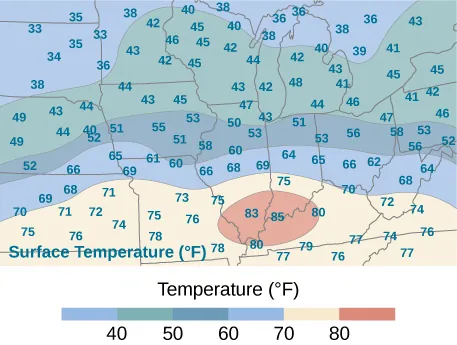Order a print copy

As an Amazon Associate we earn from qualifying purchases.